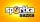# Gardens colony

Gardens colony with dimensions of 180 m and 300 m are to be completely divided into the same large squares of the highest area. Calculate how many such squares can be obtained and determine the length of the square side.

n =  15
c =  60 m

### Step-by-step explanation:Did you find an error or inaccuracy? Feel free to write us. Thank you!Tips to related online calculators
Do you want to calculate greatest common divisor two or more numbers?
Do you want to convert area units?
Do you want to convert length units?
Do you want to perform natural numbers division - find the quotient and remainder?

#### You need to know the following knowledge to solve this word math problem:

We encourage you to watch this tutorial video on this math problem:

## Related math problems and questions:

• Square gardensThe gardening colony with dimensions of 180 m and 300 m is to be completely divided into equally large square areas with the largest possible area. Calculate how many such square areas can be obtained and determine the side length of the square.
• PlumberThe plumber had to cut the metal strip with dimensions 380 cm and 60 cm to the largest squares to no waste. Calculate the length of the sides of a square. How many squares cut it?
• TilesHall has dimensions 325 &time; 170 dm. What is the largest size of square tiles that can be entire hall tiled, and how many we need them?
• Cutting paperDivide a rectangular paper with dimensions 220mm and 308mm into squares of the same size so that they are as large as possible. Specify the length of the side of the square.
• Paper squaresThe paper rectangle measuring 69 cm and 46 cm should be cut into as many squares as possible. Calculate the lengths of squares and their number.
• The landThe land, which has dimensions of 220 m and 308 m, the owner wants to divide into equally large square plots with the largest possible area. How long will one side of the plot be?
• Square tilesThe room has dimensions of 12 meters and 5.6 meters. Determine the number of square tiles and their largest dimension to exactly cover the floor.
• PexesoDrawing paper has dimensions of 60cm and 840mm. Pupils have to divide it into squares so that they can make a pexeso. What dimension must squares have if their side is to be larger than 3cm and less than 10cm?
• Largest squaresHow many of the largest square sheets did the plumber cut the honeycomb from 16 dm and 96 dm?
• Glass panelA rectangular glass panel with dimensions of 72 cm and 96 cm will cut the glazier on the largest square possible. What is the length of the side of each square? How many squares does the glazier cut?
• Argicultural fieldThe field has a rectangular shape with a width of 180 m and a circumference of 940 m. How many hectares acreage has field?
• Two hundredTwo hundred ten athletes competed in three athletics races on three fields. One hundred five athletes competed in the first, 60 in the second, and everyone else in the third. On the individual courts, the athletes were divided into groups. Each group, alt
• Reminder and quotientThere are given the number C = 281, D = 201. Find the highest natural number S so that the C:S, D:S are with the remainder of 1,1st blade 2,5 m, 2nd blade. .1.75 m. How many same long pieces of this two blades can be do the biggest? How long is one piece?At the beginning of the school year, the teacher distributed 480 workbooks and 220 textbooks. How many pupils could have the most in the classroom?Around the rectangular flowerbed with dimensions of 5.25 m and 3.50 m, roses should be planted at the same distance from each other so that the roses are located in each corner of the flower bed and are consumed as little as possible. How far do we plantIn the 6th class there are 60 girls and 72 boys. We want to divide them into groups so that the number of girls and boys is the same. How many groups can you create? How many girls will be in the group?